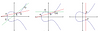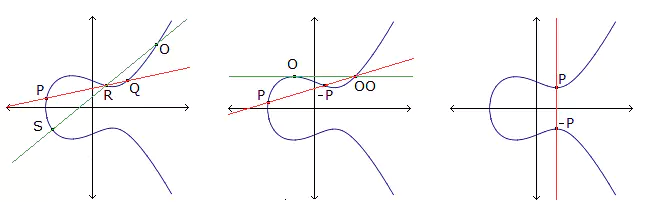# On constructions and parameters of symmetric configurations $$v_{k}$$vk

### Journal article

A. Davydov, G. Faina, M. Giulietti, S. Marcugini, F. Pambianco
Des. Codes Cryptogr., 2012

Semantic Scholar ArXiv DBLP DOI
Cite

### Cite

APA
Davydov, A., Faina, G., Giulietti, M., Marcugini, S., & Pambianco, F. (2012). On constructions and parameters of symmetric configurations $$v_{k}$$vk. Des. Codes Cryptogr.

Chicago/Turabian
Davydov, A., G. Faina, M. Giulietti, S. Marcugini, and F. Pambianco. “On Constructions and Parameters of Symmetric Configurations $$v_{k}$$Vk.” Des. Codes Cryptogr. (2012).

MLA
Davydov, A., et al. “On Constructions and Parameters of Symmetric Configurations $$v_{k}$$Vk.” Des. Codes Cryptogr., 2012.

## Abstract

The spectrum of possible parameters of symmetric configurations is investigated. We propose some new constructions, mainly based on Finite Geometry and on extension methods. New parameters are provided, both for general symmetric configurations and for cyclic symmetric configurations. For several values of $$k$$k, new upper bounds on the minimum integer $$E$$E such that for each $$v\ge E$$v≥E there exists a (cyclic) symmetric configuration $$v_{k}$$vk are obtained.

Share